﻿ 重轨星载InSAR形变监测中一种改进的大气延迟相位校正方法文章快速检索 高级检索
 大地测量与地球动力学2020, Vol. 40Issue (11): 1164-1169  DOI: 10.14075/j.jgg.2020.11.012### 引用本文ZHU Jie, LI Yu, WANG Tan, et al. An Improved Atmospheric Phase Delay Correction Method in Spaceborne Repeat-Track InSAR Monitoring[J]. Journal of Geodesy and Geodynamics, 2020, 40(11): 1164-1169.### Foundation support

Combination Project with Monitoring, Prediction and Scientific Research of Earthquake Technology, CEA, No.3JH-202001114; National Key Research and Development Program of China, No. 2018YFC1503606; Natural Science Foundation of Beijing Municipality, No. 8204077.

### 第一作者简介

ZHU Jie, assistant engineer, majors in InSAR, GNSS data processing and its application, E-mail: zhujie@seis.ac.cn.

### 文章历史

1. 中国地震台网中心，北京市三里河南横街5号，100045;
2. 首都师范大学资源环境与旅游学院，北京市西三环北路105号，100048

1 大气对InSAR测量的影响

 $\begin{array}{l} \;\;\;\;\;\;\;{\varphi _{{\rm{int}}}} = {\varphi _1} - {\varphi _2} = \\ \frac{{4{\rm{ \mathsf{ π} }}}}{\lambda }\left( {{R_1} - {R_2}} \right) + \frac{{4{\rm{ \mathsf{ π} }}}}{\lambda }\left( {\Delta {R_1} - \Delta {R_2}} \right) \end{array}$ (1)

 ${{\sigma _h} = \frac{\lambda }{{4{\rm{ \mathsf{ π} }}{B_ \bot }}}R{\rm{sin}}\theta {\sigma _\varphi }}$ (2)
 ${{\sigma _{\Delta r, 2}} = \frac{\lambda }{{4{\rm{ \mathsf{ π} }}}}{\sigma _\varphi }}$ (3)

2 空间辐射计监测大气校正方法

 ${{\rm{ZWD}} = \mathit{\Pi } \cdot {\rm{PWV}} = \frac{{{R_0}{\rho _w}\left( {\frac{{{k_3}}}{{{T_m}}} + k{' _2}} \right)}}{{{{10}^6}{M_v}}}{\rm{PWV}}}$ (4)

 ${\Delta {\varphi _{{\rm{atm}}}} = \frac{{4\pi }}{{\lambda {\rm{cos}}\theta }}\left( {{\rm{ZW}}{{\rm{D}}_{{t_1}}} - {\rm{ZW}}{{\rm{D}}_{{t_2}}}} \right) = \frac{{4{\rm{ \mathsf{ π} }}}}{\lambda }\frac{{{\rm{ZPDDM}}}}{{{\rm{cos}}\theta }}}$ (5)

3 改进的空间辐射计校正方法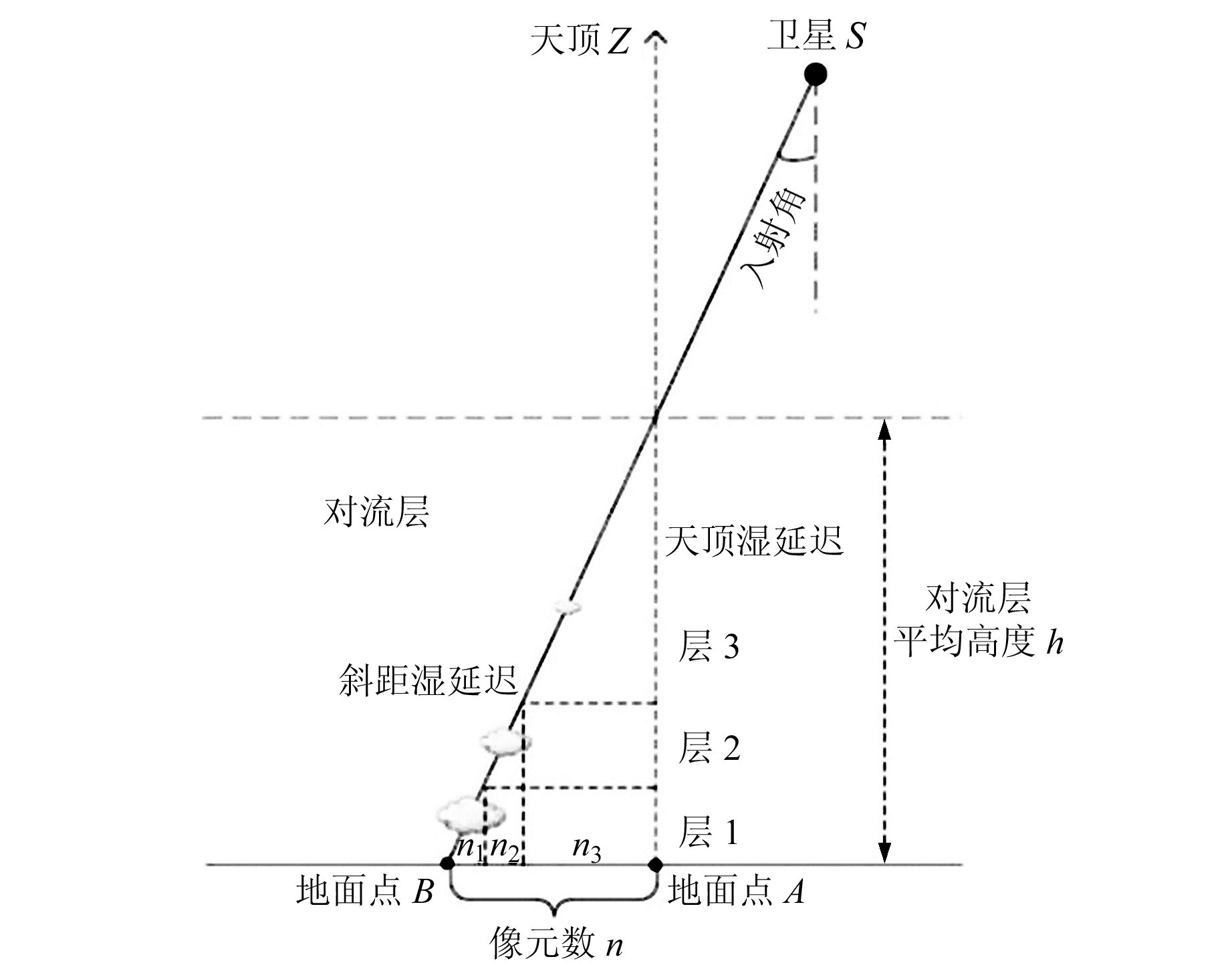图 1 天顶湿延迟与斜距湿延迟的几何关系 Fig. 1 Geometric relationship between zenith wet delays and slant wet delays

 ${{n_1} = 2{\rm{tan}}\theta /R, {n_2} = 2{\rm{tan}}\theta /R, {n_3} = 8{\rm{tan}}\theta /R}$ (6)

 ${w_i} = \left\{ \begin{array}{l} R/\left( {4{\rm{tan}}\theta } \right), \left( {i = 1, \cdots , {n_1}} \right)\\ R/\left( {8{\rm{tan}}\theta } \right), \left( {i = {n_1} + 1, \cdots , {n_1} + {n_2}} \right)\\ R/\left( {32{\rm{tan}}\theta } \right), \left( {i = {n_1} + {n_2} + 1, \cdots , n} \right) \end{array} \right.$ (7)

 $\begin{array}{l} \Delta \varphi {' _{{\rm{atm}}}} = \frac{{4\pi }}{{\lambda {\rm{cos}}\theta }}\left( {\sum\limits_{i = 1}^n {{\rm{ZPDD}}{{\rm{M}}_i} \cdot {w_i}} } \right), \\ \;\;\;\;\;\;\;\;\;\;\;\;\;\;\;\;\;\left( {i = 1, 2, \ldots , n} \right) \end{array}$ (8)

4 改进的大气校正方法验证案例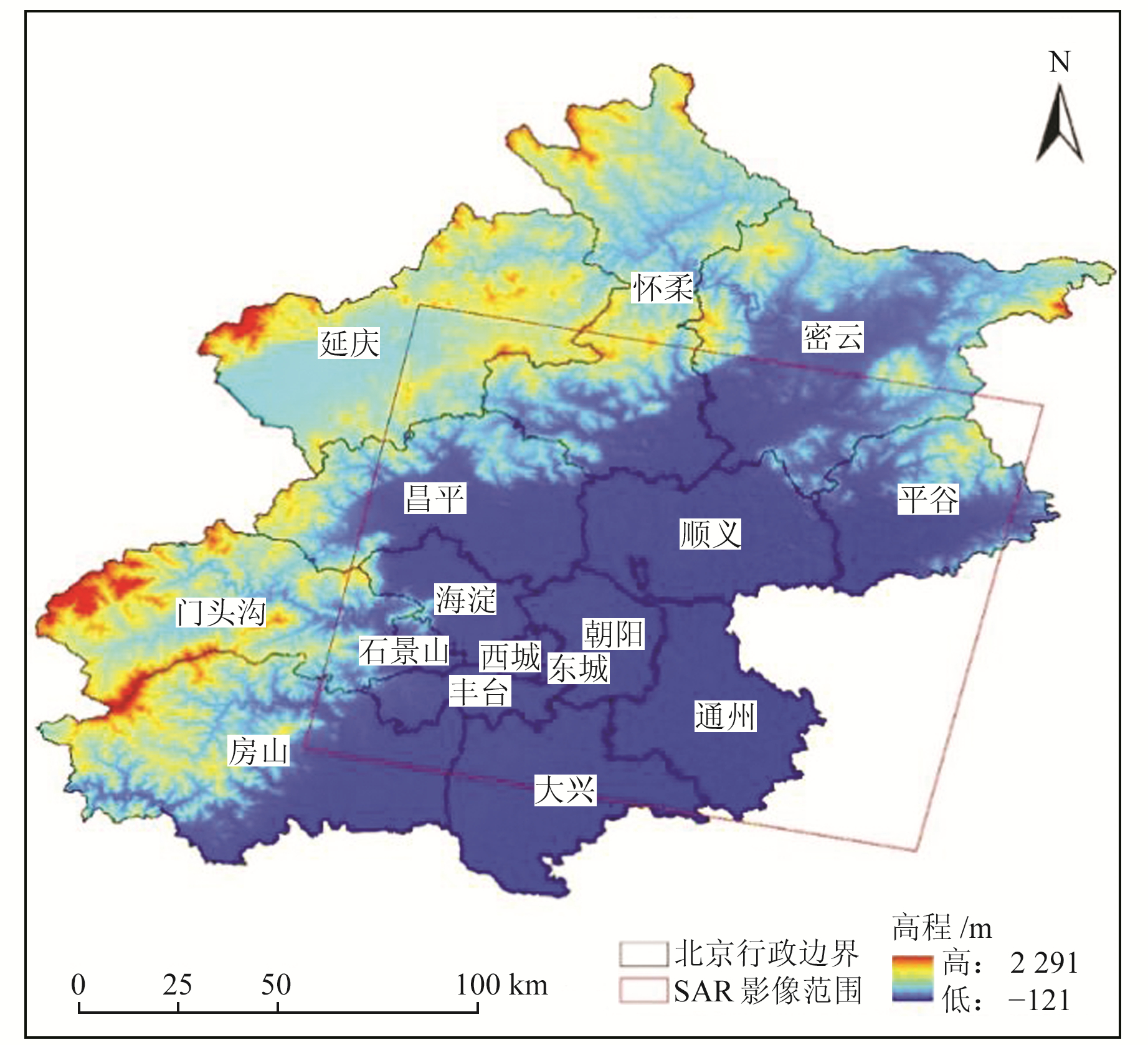图 2 ASAR影像覆盖范围 Fig. 2 ASAR image coverage
4.1 SAR数据与干涉处理表 1 Envisat ASAR数据 Tab. 1 Envisat ASAR data图 3 解缠后的干涉相位图 Fig. 3 The unwrapped interferogram
4.2 大气延迟相位误差去除表 2 MERIS数据集 Tab. 2 MERIS data

MERIS水汽反演算法对云层极其敏感，当存在云层时，MERIS反演的水汽值远小于真实值，并接近于0，如图 4(a)中黑色区域(云污染像素)所示。MERIS可提供用于识别水汽产品中云污染像素的云掩膜数据，本文借助云掩膜数据剔除MERIS水汽图中的云污染像素，并对剔除后的空像元值进行空间内插。为避免MERIS数据二次插值的误差累积，直接用含有云污染像素的MERIS水汽产品计算天顶湿延迟差，再对天顶湿延迟差进行单次内插，获得云污染像素填补后的天顶湿延迟差(图 5)。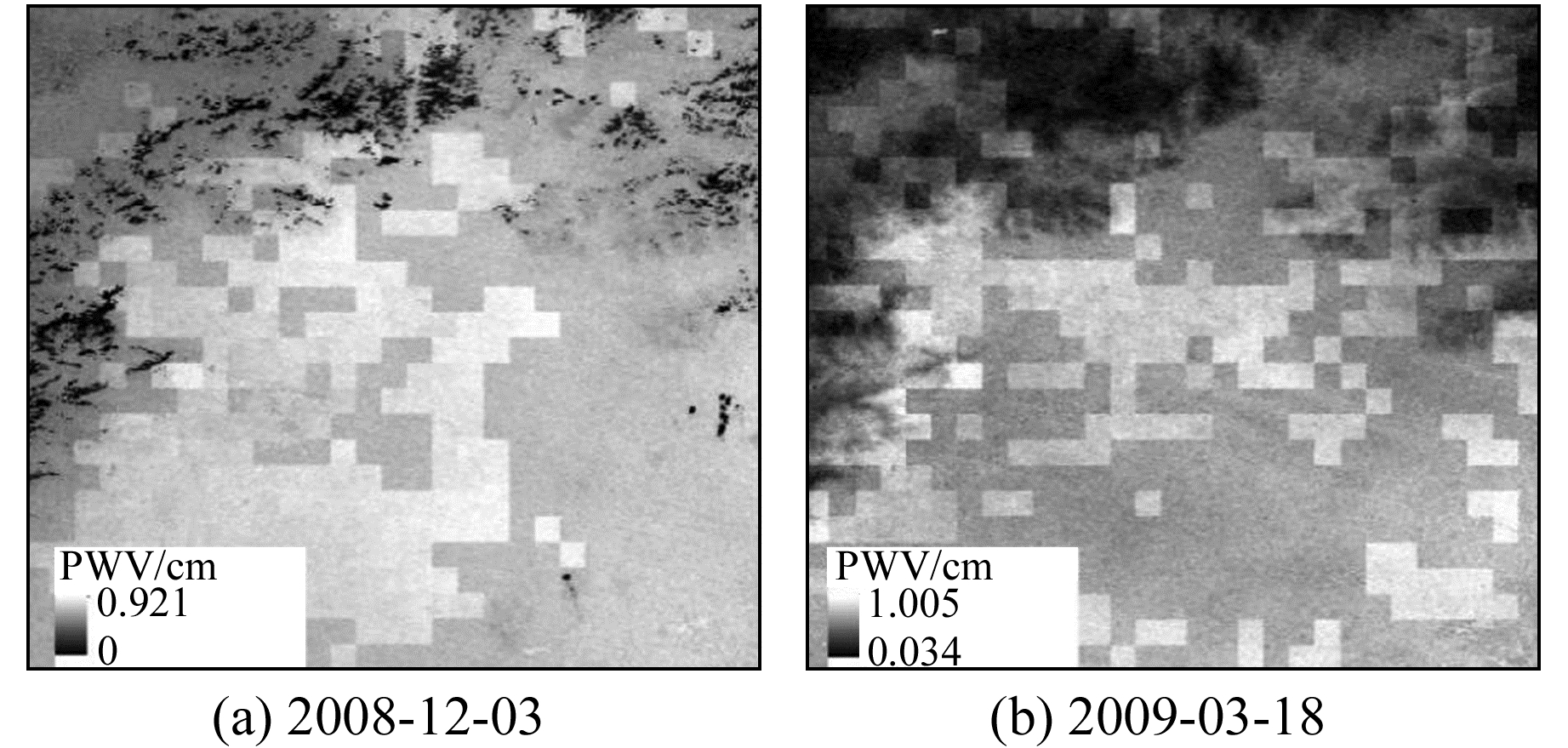图 4 MERIS可降水汽产品分布 Fig. 4 The distribution of MERIS PWV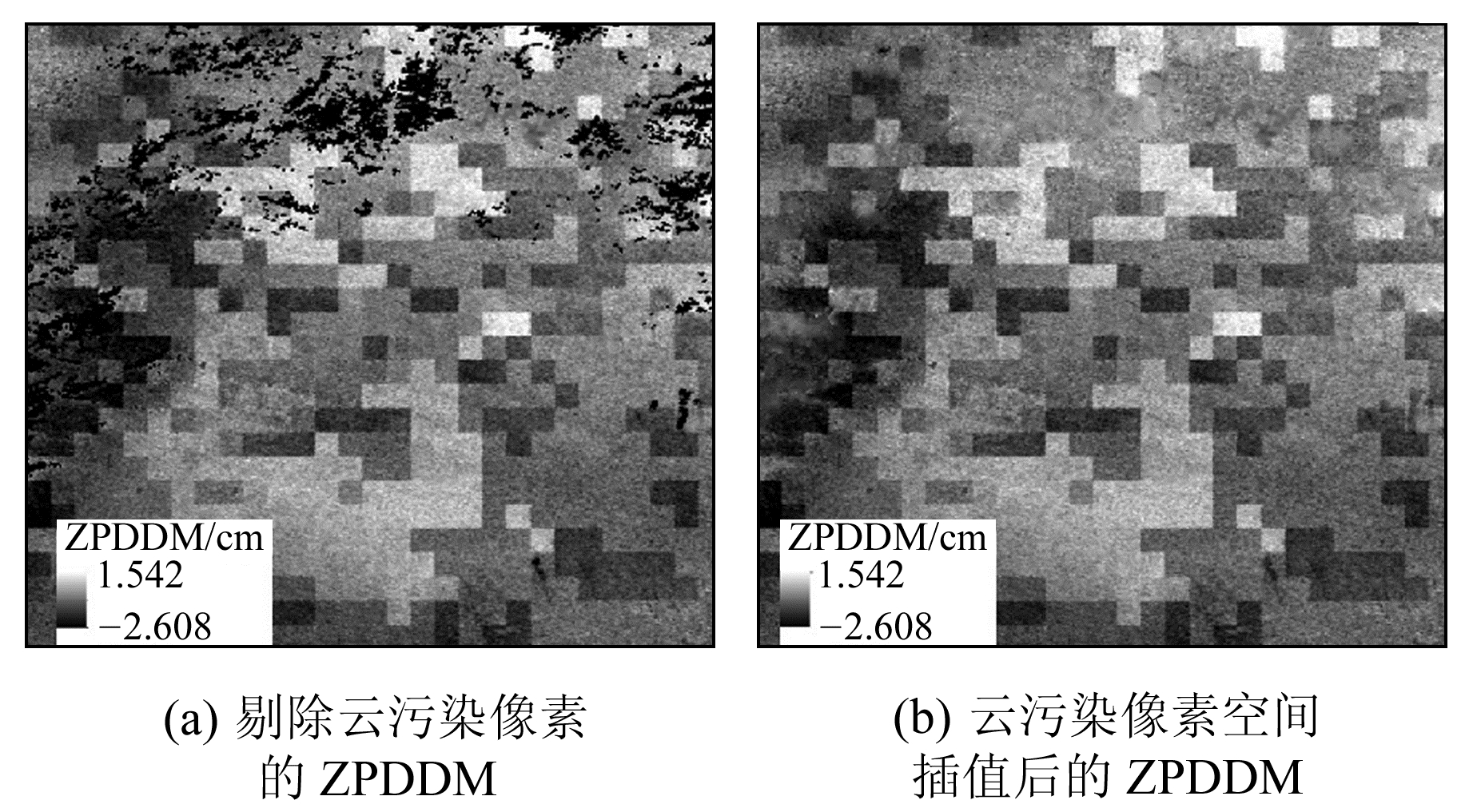图 5 天顶湿延迟差分图分布 Fig. 5 The distribution of ZPDDM

4.3 地面沉降监测结果对比与精度验证图 6 3种方法提取的地面沉降结果对比 Fig. 6 Comparison of ground subsidence field extracted by three methods表 3 InSAR监测结果与GNSS监测结果对比 Tab. 3 Comparison of InSAR monitoring results with GNSS monitoring results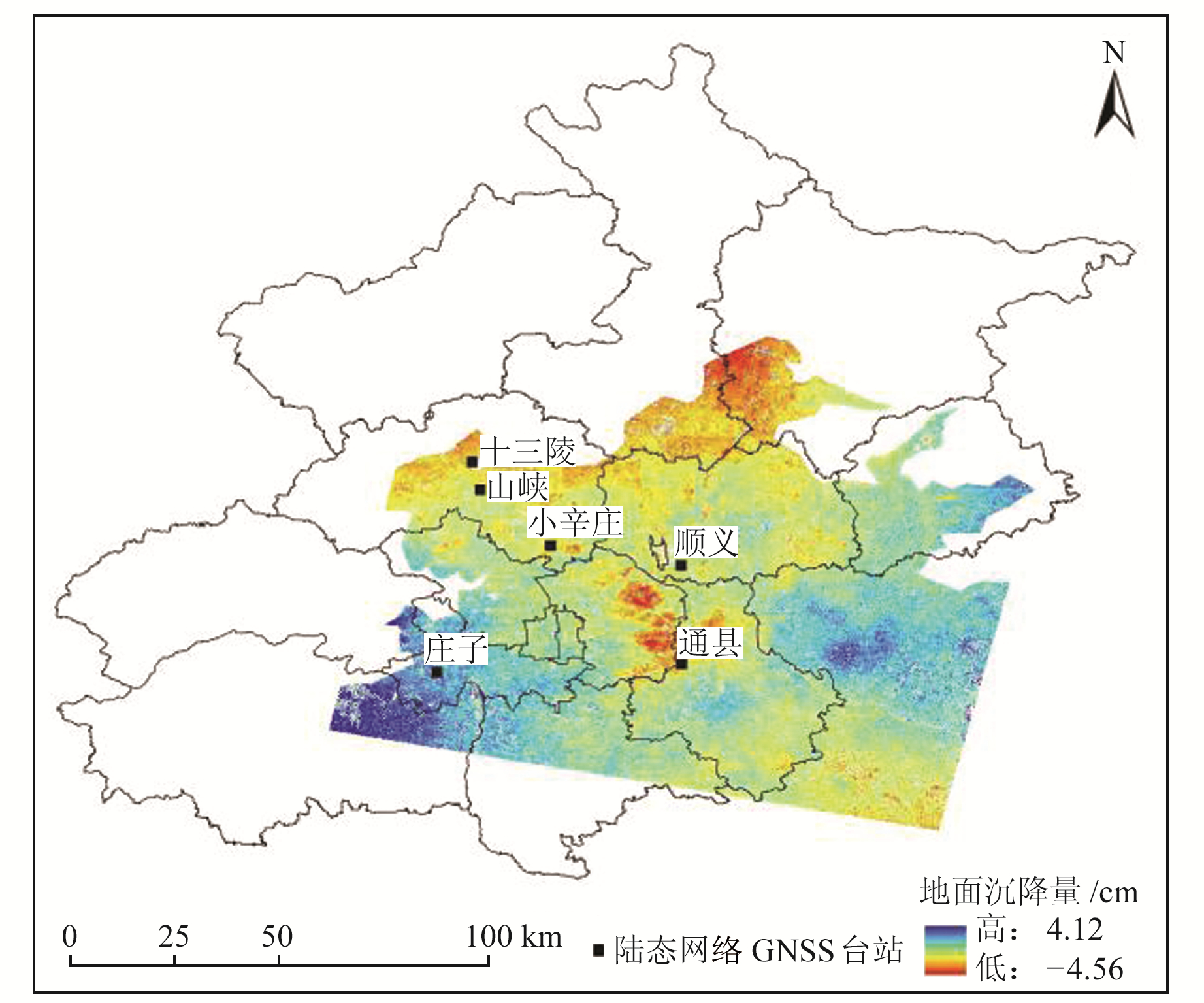图 7 地面沉降结果验证 Fig. 7 Verification of ground subsidence results
5 结语

1) 利用覆盖北京地区的ASAR数据和MERIS水汽产品，可成功削弱ASAR干涉图中的大气延迟相位，证明本文提出的方法能有效校正重轨InSAR监测中的大气误差。

2) InSAR监测结果与GNSS台站监测结果对比表明，改进的大气校正方法的均方根误差最小，为0.388 cm，表明改进的大气校正方法具有更高的地表形变监测精度。

3) 2008-12~2009-03北京地区出现不均匀地面沉降现象，朝阳和通州部分地区存在明显的沉降漏斗，最大沉降量达到4.5 cm。

  Goldstein R M. Atmospheric Limitations to Repeat-Track Radar Interferometry[J]. Geophysical Research Letters, 1995, 22(18): 2517-2520 DOI:10.1029/95GL02475 (0)  游新兆, 王琪, 乔学军, 等. 大气折射对InSAR影响的定量分析[J]. 大地测量与地球动力学, 2003, 23(2): 81-87 (You Xinzhao, Wang Qi, Qiao Xuejun, et al. Quantitative Estimation of Effect of Atmospheric Refraction on InSAR[J]. Journal of Geodesy and Geodynamics, 2003, 23(2): 81-87) (0)  Massonnet D, Feigl K, Rossi M, et al. Radar Interferometric Mapping of Deformation in the Year after the Landers Earthquake[J]. Nature, 1994, 369(6477): 227-230 DOI:10.1038/369227a0 (0)  Sandwell D T, Price E J. Phase Gradient Approach to Stacking Interferograms[J]. Journal of Geophysical Research:Solid Earth, 1998, 103(B12): 30183-30204 DOI:10.1029/1998JB900008 (0)  Ferretti A, Prati C, Rocca F. Permanent Scatters in SAR Interferometry[J]. IEEE Transactions on Geoscience and Remote Sensing, 2001, 39(1): 8-20 DOI:10.1109/36.898661 (0)  Yu C, Li Z H, Penna N T. Interferometric Synthetic Aperture Radar Atmospheric Correction Using a GPS-Based Iterative Tropospheric Decomposition Model[J]. Remote Sensing of Environment, 2018, 204: 109-121 DOI:10.1016/j.rse.2017.10.038 (0)  Tang W, Liao M S, Yuan P. Atmospheric Correction in Time-Series SAR Interferometry for Land Surface Deformation Mapping——A Case Study of Taiyuan, China[J]. Advances in Space Research, 2016, 58(3): 310-325 DOI:10.1016/j.asr.2016.05.003 (0)  Zeng Q M, Li Y, Li X F. Correction of Tropospheric Water Vapour Effect on ASAR Interferogram Using Synchronous MERIS Data[C]. IEEE International Geoscience and Remote Sensing Symposium, Barcelona, 2007 (0)  Li Z H, Muller J P, Cross P, et al. Assessment of the Potential of MERIS Near-Infrared Water Vapour Products to Correct ASAR Interferometric Measurements[J]. International Journal of Remote Sensing, 2006, 27(2): 349-365 (0)  Hanssen R H. Radar Interferometry: Data Interpretation and Error Analysis[M]. Dordrecht: Kluwer Academic Publisher, 2001 (0)  朱邦彦.InSAR对流层延迟校正及其在地表沉降监测中的应用研究[D].武汉: 武汉大学, 2017 (Zhu Bangyan. Research on Tropospheric Delay Correction of SAR Interferometry and Its Application in Land Subsidence Monitoring[D]. Wuhan: Wuhan University, 2017) (0)  Högström U. Review of Some Basic Characteristics of the Atmospheric Surface Layer[M]//Garratt J R, Taylor P A. Boundary-Layer Meteorology 25th Anniversary Volume, 1970-1995. Dordrecht: Springer, 1996 (0)  刘晓阳, 毛节泰, 李成才, 等. 大气水汽总量的垂直分解[J]. 高原气象, 2007, 26(3): 453-459 (Liu Xiaoyang, Mao Jietai, Li Chengcai, et al. Estimating Moisture Profile Using Integrated Water Vapor[J]. Plateau Meteorology, 2007, 26(3): 453-459) (0)  Chen M, Tomás R, Li Z H, et al. Imaging Land Subsidence Induced by Groundwater Extraction in Beijing(China) Using Satellite Radar Interferometry[J]. Remote Sensing, 2016, 8(6): 468 DOI:10.3390/rs8060468 (0)  宋小刚, 李德仁, 单新建, 等. 基于GPS和MODIS的ENVISAT ASAR数据干涉测量中大气改正方法研究[J]. 地球物理学报, 2009, 52(6): 1457-1464 (Song Xiaogang, Li Deren, Shan Xinjian, et al. Correction of Atmospheric Effect in ASAR Interferogram Using GPS and MODIS Data[J]. Chinese Journal of Geophysics, 2009, 52(6): 1457-1464) (0)
An Improved Atmospheric Phase Delay Correction Method in Spaceborne Repeat-Track InSAR Monitoring
ZHU Jie1LI Yu1     WANG Tan1     CHANG Zhanqiang2     YU Wen2     HAN Yufei1     WANG Yuebing1     LIU Yangyang1
1. China Earthquake Networks Center, 5 Nanheng Street, Sanlihe, Beijing 100045, China;
2. College of Resource Environment and Tourism, Capital Normal University, 105 North-Xisanhuan Road, Beijing 100048, China
Abstract: Based on conventional space radiometer method, we integrate the vertical stratification effect of water vapor in troposphere into the correction model, and propose an improved InSAR atmospheric phase delay correction method. To validate the feasibility of the proposed method, the MERIS near-infrared water vapor product is used to reduce the atmospheric phase delay in ground subsidence InSAR monitoring in Beijing. The deformation monitoring results of GNSS stations of crustal movement observation network of China (CMONOC) are used as benchmarks to verify the monitoring accuracy of the improved atmospheric correction method. The results show that RMSE of the improved atmospheric correction method, space-based radiometer method and uncorrected InSAR monitoring results are 0.388 cm, 0.603 cm and 0.685 cm, respectively, compared with the monitoring results of CMONOC GNSS stations, which shows that the improved atmospheric correction method is with higher monitoring accuracy than uncorrected and conventional method, and can effectively reduce the atmospheric phase delay errors in SAR interferograms.
Key words: InSAR; atmospheric phase delay; MERIS; ground subsidence; CMONOC